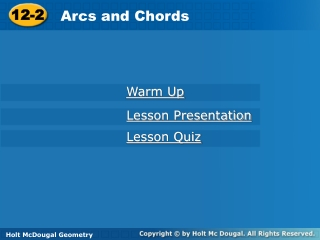DownloadDownload Presentation12-2

# 12-2

Télécharger la présentation## 12-2

- - - - - - - - - - - - - - - - - - - - - - - - - - - E N D - - - - - - - - - - - - - - - - - - - - - - - - - - -
##### Presentation Transcript

1. 12-2 Arcs and Chords Warm Up Lesson Presentation Lesson Quiz Holt McDougal Geometry Holt Geometry

2. Warm Up 1.What percent of 60 is 18? 2. What number is 44% of 6? 3. Find mWVX. 30 2.64 104.4

3. Objectives Apply properties of arcs. Apply properties of chords.

4. Vocabulary central angle semicircle arc adjacent arcs minor arc congruent arcs major arc

5. A central angleis an angle whose vertex is the center of a circle. An arcis an unbroken part of a circle consisting of two points called the endpoints and all the points on the circle between them.

6. Writing Math Minor arcs may be named by two points. Major arcs and semicircles must be named by three points.

7. mKLF = 360° – mKJF mKLF = 360° – 126° Example 1: Data Application The circle graph shows the types of grass planted in the yards of one neighborhood. Find mKLF. mKJF = 0.35(360) = 126 = 234

8. b. mAHB Check It Out! Example 1 Use the graph to find each of the following. a. mFMC mFMC = 0.30(360) = 108 Central is 30% of the . = 360° – mAMB = 0.10(360) c. mEMD mAHB =360° –0.25(360) = 36 = 270 Central is 10% of the .

9. Adjacent arcs are arcs of the same circle that intersect at exactly one point. RS and ST are adjacent arcs.

10. Find mBD. mBC = 97.4 mCD = 30.6 mBD = mBC + mCD Example 2: Using the Arc Addition Postulate Vert. s Thm. mCFD = 180 – (97.4 + 52) = 30.6 ∆Sum Thm. mCFD = 30.6 Arc Add. Post. = 97.4 + 30.6 Substitute. Simplify. = 128

11. mJKL mKL = 115° mJKL = mJK + mKL Check It Out! Example 2a Find each measure. mKPL = 180° – (40 + 25)° Arc Add. Post. = 25° + 115° Substitute. = 140° Simplify.

12. mLJN mLJN = 360° – (40 + 25)° Check It Out! Example 2b Find each measure. = 295°

13. Within a circle or congruent circles, congruent arcs are two arcs that have the same measure. In the figure STUV.

14. TV WS. Find mWS. TV  WS mTV = mWS mWS = 7(11) + 11 Example 3A: Applying Congruent Angles, Arcs, and Chords  chords have arcs. Def. of arcs 9n – 11 = 7n + 11 Substitute the given measures. 2n = 22 Subtract 7n and add 11 to both sides. n = 11 Divide both sides by 2. Substitute 11 for n. = 88° Simplify.

15. GD  NM GD NM Example 3B: Applying Congruent Angles, Arcs, and Chords C  J, andmGCD  mNJM. Find NM. GCD NJM  arcs have chords. GD = NM Def. of chords

16. Example 3B Continued C  J, andmGCD  mNJM. Find NM. 14t – 26 = 5t + 1 Substitute the given measures. 9t = 27 Subtract 5t and add 26 to both sides. t = 3 Divide both sides by 9. NM = 5(3) + 1 Substitute 3 for t. = 16 Simplify.

17. PT bisects RPS. Find RT. mRT  mTS Check It Out! Example 3a RPT  SPT RT = TS 6x = 20 – 4x 10x = 20 Add 4x to both sides. x = 2 Divide both sides by 10. RT = 6(2) Substitute 2 for x. RT = 12 Simplify.

18. mCD = mEF mCD = 100 Check It Out! Example 3b Find each measure. A B, and CD EF. Find mCD.  chords have arcs. Substitute. 25y = (30y – 20) Subtract 25y from both sides. Add 20 to both sides. 20 = 5y 4 = y Divide both sides by 5. CD = 25(4) Substitute 4 for y. Simplify.

19. Step 1 Draw radius RN. RM NP , so RM bisects NP. Example 4: Using Radii and Chords Find NP. RN = 17 Radii of a are . Step 2 Use the Pythagorean Theorem. SN2 + RS2 = RN2 SN2 + 82 = 172 Substitute 8 for RS and 17 for RN. SN2 = 225 Subtract 82 from both sides. SN= 15 Take the square root of both sides. Step 3 Find NP. NP= 2(15) = 30

20. Step 1 Draw radius PQ. PS QR , so PS bisects QR. Check It Out! Example 4 Find QR to the nearest tenth. PQ = 20 Radii of a are . Step 2 Use the Pythagorean Theorem. TQ2 + PT2 = PQ2 TQ2 + 102 = 202 Substitute 10 for PT and 20 for PQ. TQ2 = 300 Subtract 102from both sides. TQ 17.3 Take the square root of both sides. Step 3 Find QR. QR= 2(17.3) = 34.6

21. Lesson Quiz: Part I 1. The circle graph shows the types of cuisine available in a city. Find mTRQ. 158.4

22. Lesson Quiz: Part II Find each measure. 2. NGH 139 3. HL 21

23. Lesson Quiz: Part III 4. T U, and AC = 47.2. Find PL to the nearest tenth.  12.9